Email us to get an instant 20% discount on highly effective K-12 Math & English kwizNET Programs!

#### Online Quiz (WorksheetABCD)

Questions Per Quiz = 2 4 6 8 10

### High School Mathematics - 28.9 Touching Circles, Tangents

 Theorem: If two Circles touch each other internally or externally, the point of contact and the centers of the circles are collinear. Data: Two circles with centers A and B touch each other externally at point P (Figure on the left) or internally. To prove: A, B and P are collinear Construction: Draw the common tangent RPQ at P. Join AP and BP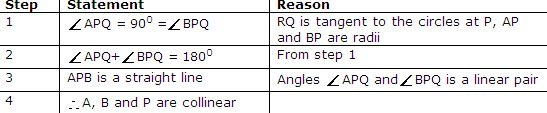For internally touching circles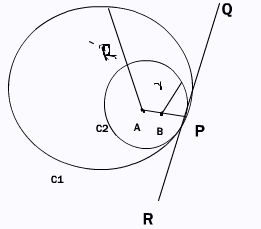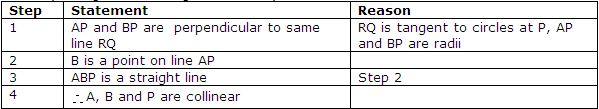Theorem: The tangents drawn to a circle from an external point are Equal Equally inclined to the line joining the external point and the center Subtend equal angles at the center Data: PA and PB are tangents from P to the circle with origin at O To Prove : PA=PB APO= BPO AOP= BOP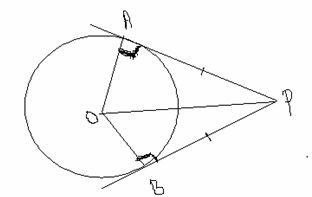Proof: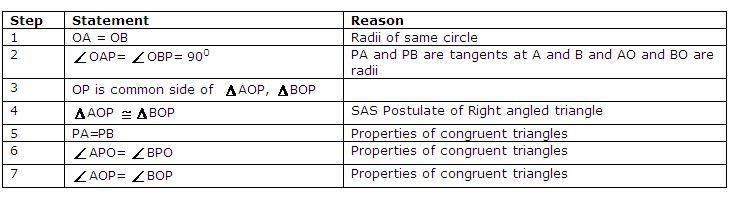Example: In the figure, XY and PC are common tangents to 2 touching circles. Prove that angle XPY = 90oTheorem: If a chord(AB) and a tangent(PT) intersect externally, then the product of lengths of the segments of the chord (PA.PB) is equal to the square of the length of the tangent(PT2)from the point of contact(T) to the point of intersection (P). Given: PT is tangent,AB is chord. To prove: PA.PB = PT2 Construction: Join O to the mid point M of AB, Join OA.Directions: Solve the following.